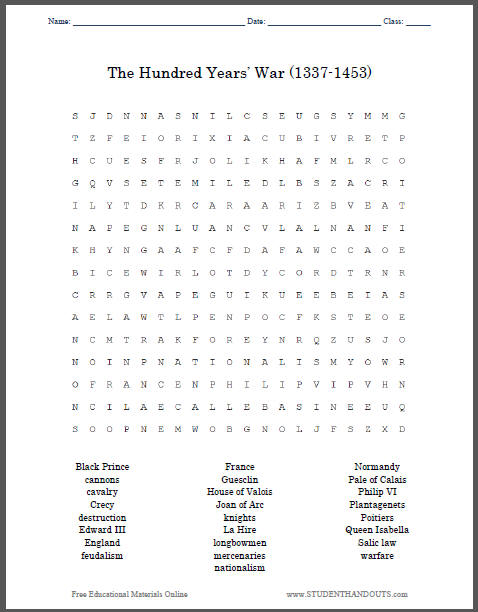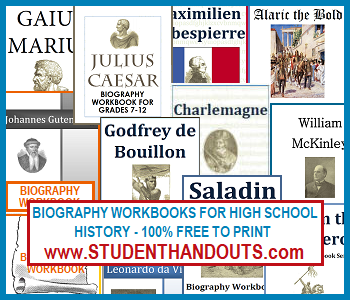﻿
The Hundred Years' War - Word Search Puzzle | Student Handouts
 The Hundred Years' War
 Free to Print - World History > Rise of Nation-States > Rise of Nation-States WorksheetsTerms and Names: Black Prince; cannons; cavalry; Crecy; destruction; Edward III; England; feudalism; France; Guesclin; House of Valois; Joan of Arc; knights; La Hire; longbowmen; mercenaries; nationalism; Normandy; Pale of Calais; Philip VI; Plantagenets; Poitiers; Queen Isabella; Salic law; warfare. Click here to print.S + D + N + S N I L C S E U G S Y M + +
T + + E + O + I + I A + + + I + R E + P
H + + + S + R + O L I + + A + M L R C O
G + + S + T + M I L E D L + S + A C R I
I L + T D + R C A R A A R I + B V E A T
N A + E + N L U A N C V L A L + A N F I
K H + N + A A F C F D A F A W C C A O E
+ I + E W + R L O T D Y C O R D + R N R
C R + G + A + E G U I K + E E + E I A S
A E + A W + L + E N P O C + + S + E O +
N + + T + A + F + R E Y N + + + U S J +
N + + N P N A T I O N A L I S M + O + +
O F R A N C E N P H I L I P V I + + H +
N + + L + + C A L L E B A S I N E E U Q
S + + P N E M W O B G N O L + + + + + +